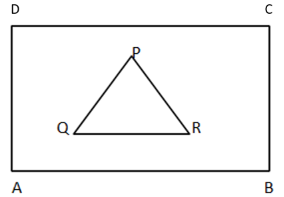A lawn in the garden is in the shape of the equilateral triangle. If the length of one of the sides of an equilateral triangle is $75m$. Find the fencing rate at $Rs$$12 per meter.Verified 150.3k+ views Hint: Find out the perimeter of the garden which is in the form of an equilateral triangle and the perimeter of the triangle is equal to the sum of all the sides and hence you can easily find the rate of fencing. Complete step-by-step answer: Here, according to the question, it is given that a lawn in the garden is in the shape of the equilateral triangle and the length of one of the sides of an equilateral triangle is 75m. It is also given that the rate of fencing 1meter is Rs$$12$. So we have to find the amount required for fencing around the triangle.
Let us draw the diagram of the garden and the lawn.Here $ABCD$ is the garden and $PQR$ is the lawn in the form of the equilateral triangle.
We have to do the fencing around this lawn or the triangle.
First of all we should know what fencing means. Fencing means to cover the lawn from all three sides.
So we can find the perimeter of the lawn and then find the rate of fencing as the rate of fencing $1m$ is given.
Perimeter$=$ sum of all sides of the triangle.
Here as it is given that it is an equilateral triangle and therefore all sides will be equal.
So, the perimeter of the triangle$=$ sum of all sides.
$= 75 + 75 + 75 = 225m$
The fencing rate is at $Rs$$12$ per $meter$.
Therefore total cost of fencing$= 12 \times 225 = 2700$
Hence the total cost is $Rs2700$.

Note: If we are asked the cost of fencing is $Rs12$ per $meter$. Here its unit is $meter$and the perimeter is also in $meter$. If it is asked $Rs12$ per $mete{r^2}$then we have to find the area as its area’s unit. So you can use this trick to remove this confusion.